# Mensturation (Mathematics) Class 6 - NCERT Questions

Q 1.

Find the perimeter of each of the following figures :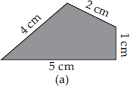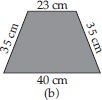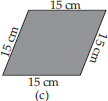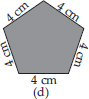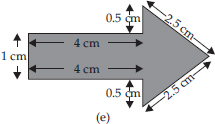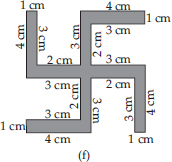SOLUTION:

(A) Perimeter = Sum of all the sides
= 4 cm + 2 cm + 1 cm + 5 cm = 12 cm
(B) Perimeter = Sum of all the sides
= 23 cm + 35 cm + 40 cm + 35 cm = 133 cm
(C) Perimeter = Sum of all the sides
= 15 cm + 15 cm + 15 cm + 15 cm = 60 cm
(D) Perimeter = Sum of all the sides
= 4 cm + 4 cm + 4 cm + 4 cm + 4 cm = 20 cm
(E) Perimeter = Sum of all the sides
= 1 cm + 4 cm + 0.5 cm + 2.5 cm + 2.5 cm
+ 0.5 cm + 4 cm = 15 cm
(F) Perimeter = Sum of all the sides
= 4 cm + 1 cm + 3 cm + 2 cm + 3 cm
+ 4 cm + 1 cm + 3 cm + 2 cm + 3 cm + 4 cm
+ 1 cm + 3 cm + 2 cm + 3 cm + 4 cm
+ 1 cm + 3 cm + 2 cm + 3 cm
= 52 cm

Q 2.

The lid of a rectangular box of sides 40 cm by 10 cm is sealed all round with tape. What is the length of the tape required?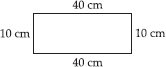SOLUTION:

Total length of tape required
= Perimeter of rectangle
= 2 × (length + breadth)
= 2 × (40 + 10) cm
= 2 × 50 cm = 100 cm = 1 m
Thus, the total length of tape required is 100 cm or 1 m.

Q 3.

A table-top measures 2 m 25 cm by 1 m 50 cm. What is the perimeter of the table-top?

SOLUTION:

Length of table-top = 2 m 25 cm = 2.25 m
Breadth of table-top = 1 m 50 cm = 1.50 m
Perimeter of table-top = 2 × (length + breadth)
= 2 × (2.25 + 1.50) m = 2 × 3.75 m = 7.50 m
Thus, perimeter of table-top is 7.5 m.

Q 4.

What is the length of the wooden strip required to frame a photograph of length and breadth
32 cm and 21 cm respectively?

SOLUTION:

Length of wooden strip
= Perimeter of photograph
= 2 × (length + breadth)
= 2 × (32 + 21) cm = 2 × 53 cm = 106 cm
Thus, the length of the wooden strip required is 106 cm.

Q 5.

A rectangular piece of land measures 0.7 km by 0.5 km. Each side is to be fenced with 4 rows of wires. What is the length of the wire needed?

SOLUTION:

Since, 4 rows of wires are needed. Therefore, the total length of wire is equal to 4 times the perimeter of land.
Perimeter of land = 2 × (length + breadth)
= 2 × (0.7 + 0.5) km = (2 × 1.2) km = 2.4 km
= 2.4 × 1000 m = 2400 m
Thus, the length of wire
= 4 × 2400 m = 9600 m = 9.6 km

Q 6.

Find the perimeter of each of the following shapes :
(A)A triangle of sides 3 cm, 4 cm and 5 cm.
(B)An equilateral triangle of side 9 cm.
(C)An isosceles triangle with equal sides 8 cm each and third side 6 cm.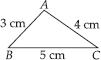SOLUTION:

(A) Perimeter of ΔABC
= AB + BC + CA = 3 cm + 5 cm + 4 cm
= 12 cm
(B) Perimeter of equilateral ΔABC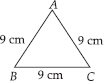= 3 × side
= 3 × 9 cm
= 27 cm
(C) Perimeter of ΔABC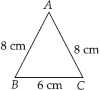= AB + BC + CA
= 8 cm + 6 cm + 8 cm
= 22 cm

Q 7.

Find the perimeter of a triangle with sides measuring 10 cm, 14 cm and 15 cm.

SOLUTION:

Perimeter of triangle
= Sum of all three sides
= 10 cm + 14 cm + 15 cm = 39 cm
Thus, perimeter of triangle is 39 cm.

Q 8.

Find the perimeter of a regular hexagon with each side measuring 8 m.

SOLUTION:

Perimeter of regular hexagon
= 6 × length of one side = 6 × 8 m = 48 m
Thus, the perimeter of regular hexagon is 48 m.

Q 9.

Find the side of the square whose perimeter is 20 m.

SOLUTION:

Perimeter of square = 4 × side
⇒ 20 m = 4 × side ⇒ side =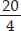m = 5m
Thus, the side of square is 5 m.

Q 10.

The perimeter of a regular pentagon is 100 cm. How long is its each side?

SOLUTION:

Perimeter of regular pentagon = 5 × side
⇒ 100 cm = 5 × side ⇒ side =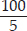cm = 20 cm
Thus, the side of regular pentagon is 20 cm.

Q 11.

A piece of string is 30 cm long. What will be the length of each side if the string is used to form:
(A) a square?
(B) an equilateral triangle?
(C) a regular hexagon?

SOLUTION:

Length of string = Perimeter of each shape
(A) Perimeter of square = 4 × side
⇒ 30 cm = 4 × side ⇒ side =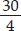cm = 7.5 cm
Thus, the length of each side of square will be 7.5 cm.
(B) Perimeter of equilateral triangle = 3 × side
⇒ 30 cm = 3 × side ⇒ side =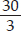cm = 10 cm
Thus, the length of each side of equilateral triangle will be 10 cm.
(C) Perimeter of regular hexagon = 6 × side
⇒ 30 cm = 6 × side ⇒ side =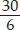cm = 5 cm
Thus, the length of each side of regular hexagon will be 5 cm.

Q 12.

Two sides of a triangle are 12 cm and 14 cm. The perimeter of the triangle is 36 cm. What is its third side?

SOLUTION:

Let the length of third side be x cm.
Length of other two sides are 12 cm and 14 cm.
Now, perimeter of triangle = 36 cm
⇒ 12 + 14 + x = 36 ⇒ 26 + x = 36
x = 36 - 26 ⇒ x = 10
Thus, the length of third side is 10 cm.

Q 13.

Find the cost of fencing a square park of side 250 m at the rate of Rs 20 per metre.

SOLUTION:

Side of square park = 250 m
Perimeter of square park = 4 × side
= 4 × 250 m = 1000 m
Since, cost of fencing for 1 metre = Rs 20
Therefore, cost of fencing for 1000 metres
= Rs 20 × 1000 = Rs 20,000

Q 14.

Find the cost of fencing a rectangular park of length 175 m and breadth 125 m at the rate of
Rs 12 per metre.

SOLUTION:

Length of rectangular park = 175 m
Breadth of rectangular park = 125 m
Perimeter of park = 2 × (length + breadth)
= 2 × (175 + 125) m
= 2 × 300 m = 600 m
Since, cost of fencing park for 1 metre = Rs 12
Therefore, cost of fencing park for 600 m
= Rs 12 × 600 = Rs 7,200

Q 15.

Sweety runs around a square park of side 75 m. Bulbul runs around a rectangular park with length 60 m and breadth 45 m. Who covers less distance?

SOLUTION:

Distance covered by Sweety
= Perimeter of square park = 4 × side
= 4 × 75 m = 300 m
Thus, distance covered by Sweety is 300 m.
Now, distance covered by Bulbul
= Perimeter of rectangular park
= 2 × (length + breadth)
= 2 × (60 + 45) m = 2 × 105 m = 210 m
Thus, Bulbul covers a distance of 210 m.
So, Bulbul covers less distance.

Q 16.

What is the perimeter of each of the following figures? What do you infer from the answers?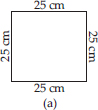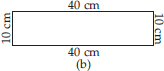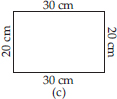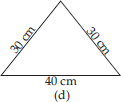SOLUTION:

(A) Perimeter of square = 4 × side
= 4 × 25 cm = 100 cm
(B) Perimeter of rectangle
= 2 × (length + breadth)
= 2 × (40 + 10) cm = 2 × 50 cm = 100 cm
(C) Perimeter of rectangle = 2 × (length + breadth)
= 2 × (30 + 20) cm = 2 × 50 cm = 100 cm
(D) Perimeter of triangle = Sum of all sides
= 30 cm + 30 cm + 40 cm = 100 cm
Thus, all the figures have same perimeter.

Q 17.

Avneet buys 9 square paving slabs, each with a side of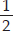m. He lays them in the form of a square.
(A) What is the perimeter of his arrangement [see fig. (i)]?
(B) Shari does not like his arrangement. She gets him to lay them out like a cross. What is the perimeter of her arrangement [see fig. (ii)]?
(C) Which has greater perimeter?
(D) Avneet wonders if there is a way of getting an even greater perimeter. Can you find a way of doing this? (The paving slabs must meet along complete edges i.e. they cannot be broken.)

SOLUTION:

(A) Side of one small square =m
∴ Side of given square =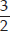m
Perimeter of square = 4 × side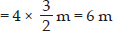(B) Perimeter of given figure
= sum of all sides = 20 ×m = 10 m
(C) The cross arrangement has greater perimeter.
(D) It is not possible to determine the arrangement with perimeter greater than 10 m.

Q 18.

Find the areas of the following figures by counting square :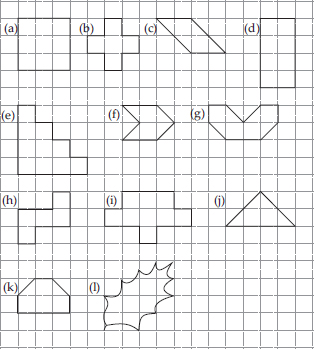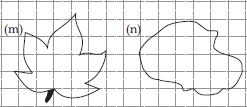SOLUTION:

(A) Number of fully-filled squares = 9
∴ Area covered by fully-filled squares
= (9 × 1) sq. units = 9 sq. units
(B) Number of fully-filled squares = 5
∴ Area covered by fully-filled squares
= (5 × 1) sq. units = 5 sq. units
(C) Number of fully-filled squares = 2
Number of half-filled squares = 4
∴ Area covered by fully-filled squares
= (2 × 1) sq. units = 2 sq. units
Area covered by half-filled squares
=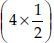sq. units = 2 sq. units
∴ Total area = (2 + 2) sq. units = 4 sq. units
(D) Number of fully-filled squares = 8
∴ Area covered by fully-filled squares
= (8 × 1) sq. units = 8 sq. units
(E) Number of fully-filled squares = 10
∴ Area covered by fully-filled squares
= (10 × 1) sq. units = 10 sq. units
(F) Number of fully-filled squares = 2
Number of half-filled squares = 4
∴ Area covered by fully-filled squares
= (2 × 1) sq. units = 2 sq. units
Area covered by half-filled squares
=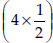sq. units = 2 sq. units
∴ Total area = (2 + 2) sq. units = 4 sq. units
(G) Number of fully-filled squares = 4
Number of half-filled squares = 4
∴ Area covered by fully-filled squares
= (4 × 1) sq. units = 4 sq. units
Area covered by half-filled squares
=sq. units = 2 sq. units
∴ Total area = (4 + 2) sq. units = 6 sq. units
(h) Number of fully-filled squares = 5
∴ Area covered by fully-filled squares
= (5 × 1) sq. units = 5 sq. units
(i) Number of fully-filled squares = 9
∴ Area covered by fully-filled squares
= (9 × 1) sq. units = 9 sq. units
(j) Number of fully-filled squares = 2
Number of half-filled squares = 4
∴ Area covered by fully-filled squares
= (2 × 1) sq. units = 2 sq. units
Area covered by half-filled squares
=sq. units = 2 sq. units
∴ Total area = (2 + 2) sq. units = 4 sq. units
(k) Number of fully-filled squares = 4
Number of half-filled squares = 2
∴ Area covered by fully-filled squares
= (4 × 1) sq. units = 4 sq. unit
Area covered by half-filled squares
=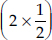sq. units = 1 sq. units
∴ Total area = (4 + 1) sq. units = 5 sq. units
(l) Number of fully-filled squares = 2,
Number of half-filled squares = 0,
Number of more than half-filled squares = 6
and number of less than half-filled squares = 6.
Now, estimated area covered by
fully-filled squares = 2 sq. units,
half filled squares = 0 sq. units
more than half-filled squares = 6 sq. units
and less than half-filled squares = 0 sq. unit
∴ Total area = (2 + 0 + 6 + 0) sq. units = 8 sq. units.
(m) Number of fully-filled squares = 5
Number of more than half-filled squares =9
and number of less than half-filled squares = 12
Estimated area covered by
fully-filled squares = 5 sq. units
more than half-filled squares = 9 sq. units
and less than half-filled squares = 0 sq. unit
∴ Total area = (5 + 9+ 0) sq. units = 14 sq. units
(n) Number of fully-filled squares = 8
Number of more than half-filled squares = 10
and number of less than half-filled squares = 9
Estimated area covered by
fully-filled squares = 8 sq. units,
more than half-filled squares = 10 sq. units
less than half-filled squares = 0 sq. unit
∴ Total area = (8 + 10 + 0) sq. units = 18 sq. units.

Q 19.

Find the areas of the rectangles whose sides are :
(A) 3 cm and 4 cm
(B) 12 m and 21 m
(C) 2 km and 3 km
(D) 2 m and 70 cm

SOLUTION:

(A) Area of rectangle = length × breadth
= 3 cm × 4 cm = 12 cm2
(B) Area of rectangle = length × breadth
= 12 m × 21 m = 252 m2
(C) Area of rectangle = length × breadth
= 2 km × 3 km = 6 km2
(D) Area of rectangle = length × breadth
= 2 m × 70 cm = 2 m × 0.7 m = 1.4 m2

Q 20.

Find the areas of the squares whose sides are:
(A) 10 cm
(B) 14 cm
(C) 5 m

SOLUTION:

(A) Area of square = side × side
= 10 cm × 10 cm = 100 cm2
(B) Area of square = side × side
= 14 cm × 14 cm = 196 cm2
(C) Area of square = side × side
= 5 m × 5 m = 25 m2

Q 21.

The length and breadth of three rectangles are as given below :
(A) 9 m and 6 m
(B) 17 m and 3 m
(C) 4 m and 14 m
Which one has the largest area and which one has the smallest?

SOLUTION:

(A) Area of rectangle = length × breadth
= 9 m × 6 m = 54 m2
(B) Area of rectangle = length × breadth
= 17 m × 3 m = 51 m2
(C) Area of rectangle = length × breadth
= 4 m × 14 m = 56 m2
Thus, rectangle (C) has the largest area, i.e. 56 m2 and rectangle (B) has the smallest area, i.e., 51 m2.

Q 22.

The area of a rectangular garden 50 m long is
300 sq m. Find the width of the garden.

SOLUTION:

Length of rectangle = 50 m
Area of rectangle = 300 m2
Since, area of rectangle = length × breadth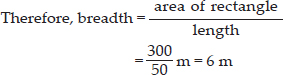Thus, the breadth of the garden is 6 m.

Q 23.

What is the cost of tiling a rectangular plot of land 500 m long and 200 m wide at the rate of
Rs 8 per hundred sq. m?

SOLUTION:

Length of land = 500 m
breadth of land = 200 m
Area of land = length × breadth
= 500 m × 200 m = 1,00,000 sq. m
Cost of tiling 100 sq. m of land = Rs 8
∴ Cost of tiling 1,00,000 sq. m of land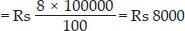Q 24.

A table-top measures 2 m by 1 m 50 cm. What is its area in square metres?

SOLUTION:

Length of table-top = 2 m
Breadth of table-top = 1 m 50 cm = 1.50 m
∴ Area of table-top = length × breadth
= 2 m × 1.50 m = 3 m2

Q 25.

A room is 4 m long and 3 m 50 cm wide. How many square metres of carpet is needed to cover the floor of the room?

SOLUTION:

Length of room = 4 m
And breadth of room = 3 m 50 cm = 3.50 m
∴ Area of carpet = length × breadth
= 4 m × 3.50 m = 14 m2

Q 26.

A floor is 5 m long and 4 m wide. A square carpet of sides 3 m is laid on the floor. Find the area of the floor that is not carpeted.

SOLUTION:

Length of floor = 5 m
and breadth of floor = 4 m
Area of floor = length × breadth = 5 m × 4 m = 20 m2
Now, side of square carpet = 3 m
Area of square carpet = side × side = 3 m × 3 m = 9 m2
∴ Area of floor that is not carpeted
= 20 m2 - 9 m2 = 11 m2

Q 27.

Five square flower beds each of sides 1 m are dug on a piece of land 5 m long and 4 m wide. What is the area of the remaining part of the land?

SOLUTION:

Side of square flower bed = 1 m
Area of square flower bed = side × side
= 1 m × 1 m = 1 m2
∴ Area of 5 square flower beds = (1 × 5) m2 = 5 m2
Now, length of land = 5 m
And breadth of land = 4 m
Area of land = length × breadth = 5 m × 4 m = 20 m2
∴ Area of remaining part
= Area of land - Area of 5 flower beds
= 20 m2 - 5 m2 = 15 m2

Q 28.

By splitting the following figures into rectangles, find their areas (The measures are given in centimetres).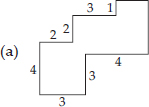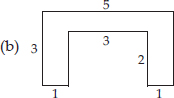SOLUTION:

(A) We have,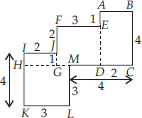Area of square HKLM = (3 × 3) cm2 = 9 cm2
Area of rectangle IJGH = (1 × 2) cm2 = 2 cm2
Area of square FEDG = (3 × 3) cm2 = 9 cm2
Area of rectangle ABCD = (2 × 4) cm2 = 8 cm2
∴ Total area of the figure
= (9 + 2 + 9 + 8) cm2 = 28 cm2
(B) We have,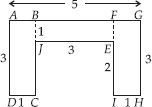Area of rectangle ABCD = (3 × 1) cm2 = 3 cm2
Area of rectangle BJEF = (3 × 1) cm2 = 3 cm2
Area of rectangle FGHI = (3 × 1) cm2 = 3 cm2
∴ Total area of the figure = (3 + 3 + 3) cm2 = 9 cm2

Q 29.

Split the following shapes into rectangles and find their areas. (The measures are given in centimetres).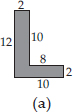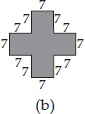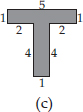SOLUTION:

(A) We have,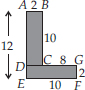Area of rectangle ABCD = (2 × 10) cm2 = 20 cm2
Area of rectangle DEFG = (10 × 2) cm2 = 20 cm2
∴ Total area of the figure = (20 + 20) cm2 = 40 cm2
(B) We have,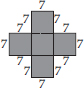There are 5 squares each of side 7 cm.
Area of one square = (7 × 7) cm2 = 49 cm2
∴ Area of 5 squares = (5 × 49) cm2 = 245 cm2
(C) We have,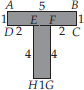Area of rectangle ABCD = (5 × 1) cm2 = 5 cm2
Area of rectangle EFGH = (4 × 1) cm2 = 4 cm2
∴ Total area of the figure = (5 + 4) cm2 = 9 cm2

Q 30.

How many tiles whose length and breadth are 12 cm and 5 cm respectively will be needed to fit in a rectangular region whose length and breadth are respectively:
(A) 100 cm and 144 cm
(B) 70 cm and 36 cm.

SOLUTION:

(A) Area of rectangular region
= length × breadth = 100 cm × 144 cm = 14400 cm2
Area of one tile = 12 cm × 5 cm = 60 cm2
∴ Number of tiles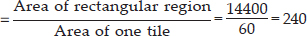Thus, 240 tiles are required.
(B) Area of rectangular region
= length × breadth = 70 cm × 36 cm = 2520 cm2
Area of one tile = 12 cm × 5 cm = 60 cm2
∴ Number of tiles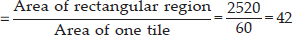Thus, 42 tiles are required.